Open in App
Not now

# Skin Cancer Detection using TensorFlow

• Last Updated : 21 Nov, 2022

In this article, we will learn how to implement a Skin Cancer Detection model using Tensorflow. We will use a dataset that contains images for the two categories that are malignant or benign. We will use the transfer learning technique to achieve better results in less amount of training. We will use EfficientNet architecture as the backbone of our model along with the pre-trained weights of the same obtained by training it on the image net dataset.

## Importing Libraries

Python libraries make it very easy for us to handle the data and perform typical and complex tasks with a single line of code.

• Pandas – This library helps to load the data frame in a 2D array format and has multiple functions to perform analysis tasks in one go.
• Numpy – Numpy arrays are very fast and can perform large computations in a very short time.
• Matplotlib – This library is used to draw visualizations.
• Sklearn – This module contains multiple libraries having pre-implemented functions to perform tasks from data preprocessing to model development and evaluation.
• Tensorflow – This is an open-source library that is used for Machine Learning and Artificial intelligence and provides a range of functions to achieve complex functionalities with single lines of code.

## Python3

 `import` `numpy as np``import` `pandas as pd``import` `seaborn as sb``import` `matplotlib.pyplot as plt`` ` `from` `glob ``import` `glob``from` `PIL ``import` `Image``from` `sklearn.model_selection ``import` `train_test_split`` ` `import` `tensorflow as tf``from` `tensorflow ``import` `keras``from` `keras ``import` `layers``from` `functools ``import` `partial`` ` `AUTO ``=` `tf.data.experimental.AUTOTUNE``import` `warnings``warnings.filterwarnings(``'ignore'``)`

Now, let’s check the number of images we have got here. You can download the image dataset from here.

## Python3

 `images ``=` `glob(``'train/*/*.jpg'``)``len``(images)`

Output:

`2637`

## Python3

 `df ``=` `pd.DataFrame({``'filepath'``: images})``df[``'label'``] ``=` `df[``'filepath'``].``str``.split(``'/'``, expand``=``True``)[``1``]``df.head()`

Output: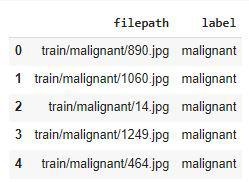Converting the labels to 0 and 1 will save our work of label encoding so, let’s do this right now.

## Python3

 `df[``'label_bin'``] ``=` `np.where(df[``'label'``].values ``=``=` `'malignant'``, ``1``, ``0``)``df.head()`

Output: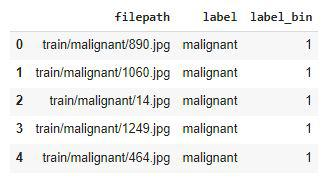## Python3

 `x ``=` `df[``'label'``].value_counts()``plt.pie(x.values,``        ``labels``=``x.index,``        ``autopct``=``'%1.1f%%'``)``plt.show()`

Output: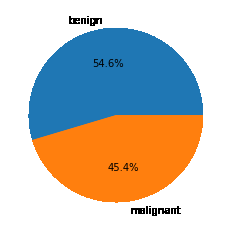An approximately equal number of images have been given for each of the classes so, data imbalance is not a problem here.

## Python3

 `for` `cat ``in` `df[``'label'``].unique():``    ``temp ``=` `df[df[``'label'``] ``=``=` `cat]`` ` `    ``index_list ``=` `temp.index``    ``fig, ax ``=` `plt.subplots(``1``, ``4``, figsize``=``(``15``, ``5``))``    ``fig.suptitle(f``'Images for {cat} category . . . .'``, fontsize``=``20``)``    ``for` `i ``in` `range``(``4``):``        ``index ``=` `np.random.randint(``0``, ``len``(index_list))``        ``index ``=` `index_list[index]``        ``data ``=` `df.iloc[index]`` ` `        ``image_path ``=` `data[``0``]`` ` `        ``img ``=` `np.array(Image.``open``(image_path))``        ``ax[i].imshow(img)``plt.tight_layout()``plt.show()`

Output: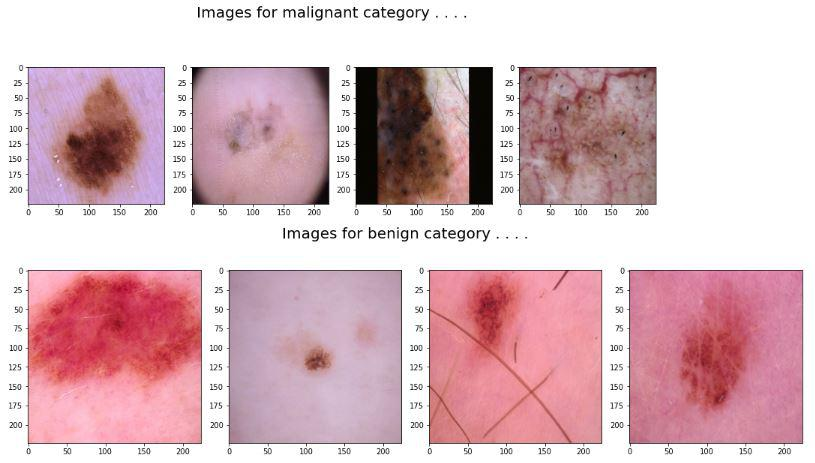Now, let’s split the data into training and validation parts by using the train_test_split function.

## Python3

 `features ``=` `df[``'filepath'``]``target ``=` `df[``'label_bin'``]`` ` `X_train, X_val,\``    ``Y_train, Y_val ``=` `train_test_split(features, target,``                                      ``test_size``=``0.15``,``                                      ``random_state``=``10``)`` ` `X_train.shape, X_val.shape`

Output:

`((2241,), (396,))`

## Python3

 `def` `decode_image(filepath, label``=``None``):`` ` `    ``img ``=` `tf.io.read_file(filepath)``    ``img ``=` `tf.image.decode_jpeg(img)``    ``img ``=` `tf.image.resize(img, [``224``, ``224``])``    ``img ``=` `tf.cast(img, tf.float32) ``/` `255.0`` ` `    ``if` `label ``=``=` `None``:``        ``return` `img`` ` `    ``return` `img, label`

Image input pipelines have been implemented below so, that we can pass them without any need to load all the data beforehand.

## Python3

 `train_ds ``=` `(``    ``tf.data.Dataset``    ``.from_tensor_slices((X_train, Y_train))``    ``.``map``(decode_image, num_parallel_calls``=``AUTO)``    ``.``map``(partial(process_data), num_parallel_calls``=``AUTO)``    ``.batch(``32``)``    ``.prefetch(AUTO)``)`` ` `val_ds ``=` `(``    ``tf.data.Dataset``    ``.from_tensor_slices((X_val, Y_val))``    ``.``map``(decode_image, num_parallel_calls``=``AUTO)``    ``.batch(``32``)``    ``.prefetch(AUTO)``)`

## Model Development

For this task, we will use the EfficientNet architecture and leverage the benefit of pre-trained weights of such large networks.

### Model Architecture

We will implement a model using the  Functional API of Keras which will contain the following parts:

• The base model is the EfficientNet model in this case.
• The Flatten layer flattens the output of the base model’s output.
• Then we will have two fully connected layers followed by the output of the flattened layer.
• We have included some BatchNormalization layers to enable stable and fast training and a Dropout layer before the final layer to avoid any possibility of overfitting.
• The final layer is the output layer which outputs soft probabilities for the three classes.

## Python3

 `from` `tensorflow.keras.applications.efficientnet ``import` `EfficientNetB7`` ` `pre_trained_model ``=` `EfficientNetB7(``    ``input_shape``=``(``224``, ``224``, ``3``),``    ``weights``=``'imagenet'``,``    ``include_top``=``False``)`` ` `for` `layer ``in` `pre_trained_model.layers:``    ``layer.trainable ``=` `False`

Output:

`258076736/258076736 [==============================] - 3s 0us/step`

## Python3

 `from` `tensorflow.keras ``import` `Model`` ` `inputs ``=` `layers.``Input``(shape``=``(``224``, ``224``, ``3``))``x ``=` `layers.Flatten()(inputs)`` ` `x ``=` `layers.Dense(``256``, activation``=``'relu'``)(x)``x ``=` `layers.BatchNormalization()(x)``x ``=` `layers.Dense(``256``, activation``=``'relu'``)(x)``x ``=` `layers.Dropout(``0.3``)(x)``x ``=` `layers.BatchNormalization()(x)``outputs ``=` `layers.Dense(``1``, activation``=``'sigmoid'``)(x)`` ` `model ``=` `Model(inputs, outputs)`

While compiling a model we provide these three essential parameters:

• optimizer – This is the method that helps to optimize the cost function by using gradient descent.
• loss – The loss function by which we monitor whether the model is improving with training or not.
• metrics – This helps to evaluate the model by predicting the training and the validation data.

## Python3

 `model.``compile``(``    ``loss``=``tf.keras.losses.BinaryCrossentropy(from_logits``=``True``),``    ``optimizer``=``'adam'``,``    ``metrics``=``[``'AUC'``]``)`

Now let’s train our model.

## Python3

 `history ``=` `model.fit(train_ds,``                    ``validation_data``=``val_ds,``                    ``epochs``=``5``,``                    ``verbose``=``1``)`

Output:

```Epoch 1/5
71/71 [==============================] - 5s 54ms/step - loss: 0.5478 - auc: 0.8139 - val_loss: 2.6825 - val_auc: 0.6711
Epoch 2/5
71/71 [==============================] - 3s 49ms/step - loss: 0.4547 - auc: 0.8674 - val_loss: 1.1363 - val_auc: 0.8328
Epoch 3/5
71/71 [==============================] - 3s 48ms/step - loss: 0.4288 - auc: 0.8824 - val_loss: 0.8702 - val_auc: 0.8385
Epoch 4/5
71/71 [==============================] - 3s 48ms/step - loss: 0.4044 - auc: 0.8933 - val_loss: 0.6367 - val_auc: 0.8561
Epoch 5/5
71/71 [==============================] - 3s 49ms/step - loss: 0.3891 - auc: 0.9019 - val_loss: 0.9296 - val_auc: 0.8558```

Let’s visualize the training and validation loss and AUC with each epoch.

## Python3

 `hist_df ``=` `pd.DataFrame(history.history)``hist_df.head()`

Output: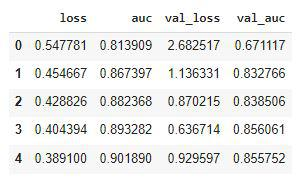## Python3

 `hist_df[``'loss'``].plot()``hist_df[``'val_loss'``].plot()``plt.title(``'Loss v/s Validation Loss'``)``plt.legend()``plt.show()`

Output: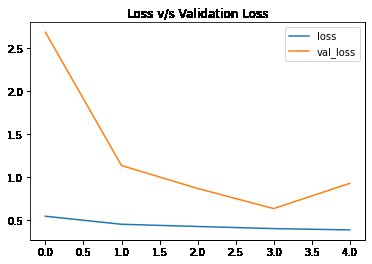Training loss has not decreased over time as much as the validation loss.

## Python3

 `hist_df[``'auc'``].plot()``hist_df[``'val_auc'``].plot()``plt.title(``'AUC v/s Validation AUC'``)``plt.legend()``plt.show()`

Output: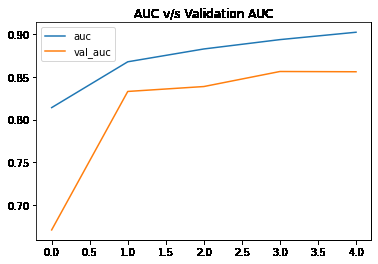My Personal Notes arrow_drop_up